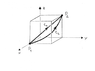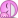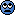# Work and line integral

• JulienB

## Homework Statement

Hi everybody! I would like to make sure I properly solved that problem because I find the result strange:

Given a force field ##F_x = axy^3##, ##F_y = bx^2y^2##, ##F_z = cz^3##.
Calculate the work with the line integral ##\int_{C} \vec{F} \cdot d\vec{r}## from point ##P_1(1,0,0)## to ##P_2(0,1,1)## along:
a) a line;
b) an helix by the z-axis.

## The Attempt at a Solution

Okay so first I parametered the line:
$$\vec{r}(\tau) = (1-\tau, \tau, \tau) \mbox{ with } \tau \in [0,1]$$
Is the first component of my vector correct? I have a little doubt about it.
$$\implies \dot{\vec{r}}(\tau) = (-1,1,1) \\ \implies A = \int_{0}^{1} \vec{F} \cdot \vec{r} \cdot d\tau \\ = \int_{0}^{1} (a (1 - \tau) \tau^3, b(1-\tau)^2\tau^2, c\tau^3)(-1,1,1)d\tau \\$$
Here again, I have a little doubt about what I did with the force field vector. Is that correct?
$$\int_{0}^{1} (-a\tau^3 + a\tau^4 + b\tau^2 - 2b\tau^3 + b\tau^4 + c\tau^3) d\tau \\ a[\frac{\tau^5}{5} - \frac{\tau^4}{4}]_0^1 + b[\frac{\tau^5}{5} + \frac{\tau^3}{3} - \frac{\tau^4}{2}]_0^1 + c[\frac{\tau^4}{4}]_0^1 \\ A = -\frac{a}{20} + \frac{b}{30} + \frac{c}{4}$$
So yeah here is my result for (a). I'd like to know if I use the right method before I attempt to solve (b).

What do you guys think?

Julien.

Last edited:
Looks good. (In your integral for A you meant to type a dot over the r vector.)

@TSny Ah yes, thanks a lot. I leave it as it is so that your message doesn't look strange in the feed :)

Great that it is correct. I'm struggling to find the equation for (b) though, I don't even get how to search for it. There is a picture with the problem, I attached it.

Thanks for all your help, I'm always making progress here.

Julien.

#### Attachments

•Photo 07-05-16 23 05 41.png
18.7 KB · Views: 307
Is the equation ##x(\tau) = \cos \tau, y(\tau) = \sin \tau, z = \tau## actually?

Is the equation ##x(\tau) = \cos \tau, y(\tau) = \sin \tau, z = \tau## actually?
Close. Make sure the path goes through the final point (0, 1, 1).

@TSny Oh yeah I said that kind of randomly but I think I get it now. What about that: ##x(\tau) = \cos( \tau), y(\tau) = \sin (\frac{\tau}{2}), z(\tau) = \sin(\frac{\tau}{2})## with ## \tau \in [0,\pi]##?

This is no longer a helix. You were much closer before.

@TSny Oops. The integral was also not that nice anymore.Okay another try: ##x(\tau) = \cos(\pi \tau), y(\tau) = sin(\frac{\pi}{2} \tau) , z(\tau) = \tau ## with this time ##\tau \in [0,1]##.

This is not a helix either and it doesn't pass through P2. But it's pretty close!

@Ah I think I was thinking too much about the path. This should be it: ##x(\tau) = cos(\frac{\pi}{2} \tau) , y(\tau) = sin(\frac{\pi}{2} \tau), z(\tau) = \tau ##, right?

@Ah I think I was thinking too much about the path. This should be it: ##x(\tau) = cos(\frac{\pi}{2} \tau) , y(\tau) = sin(\frac{\pi}{2} \tau), z(\tau) = \tau ##, right?
Right. Good luck with the integration. A little tedious. Can you use software?

@TSny I guess I could, but I'll first give it a go. See you in 20 hours(It's not THAT bad.)

•JulienB
@TSny At the end I used a calculator because I must go to sleep. Did you also calculate it? I get ##A = \frac{-a}{5} + \frac{2b}{15} + \frac{c}{4}##.

I have an extra question: in the next exercise, they ask for which values a, b, c does this force field have a potential, and what is it. I answered the first one like that:

##\vec{F} \mbox{ is conservative, if } \vec{\nabla} \times \vec{F} = 0\\
\vec{\nabla} \times \vec{F} = (\frac{\partial F_z}{\partial y} - \frac{\partial F_y}{\partial z})\vec{e_x} + (\frac{\partial F_x}{\partial x} - \frac{\partial F_z}{\partial x})\vec{e_y} + (\frac{\partial F_y}{\partial x} - \frac{\partial F_x}{\partial y})\vec{e_z} \\
= 0 \vec{e_x} + 0 \vec{e_y} + (2bxy^2 - 3axy^2) \vec{e_z} \\
= xy^2 (2b - 3a) \vec{e_z} \\
\implies \vec{F} \mbox{ is conservative, if } 2b - 3a = 0 \\
\implies b = \frac{3}{2} a##
Is that a correct answer? I'm very unexperienced with gradients. What does it mean physically that z doesn't play a role in the "conservativeness" of the field?

Thanks a lot.

Julien.

@TSny At the end I used a calculator because I must go to sleep. Did you also calculate it? I get ##A = \frac{-a}{5} + \frac{2b}{15} + \frac{c}{4}##.
Yes, that's what my computer got.
I have an extra question: in the next exercise, they ask for which values a, b, c does this force field have a potential, and what is it. I answered the first one like that:

##\vec{F} \mbox{ is conservative, if } \vec{\nabla} \times \vec{F} = 0\\
\vec{\nabla} \times \vec{F} = (\frac{\partial F_z}{\partial y} - \frac{\partial F_y}{\partial z})\vec{e_x} + (\frac{\partial F_x}{\partial x} - \frac{\partial F_z}{\partial x})\vec{e_y} + (\frac{\partial F_y}{\partial x} - \frac{\partial F_x}{\partial y})\vec{e_z} \\
= 0 \vec{e_x} + 0 \vec{e_y} + (2bxy^2 - 3axy^2) \vec{e_z} \\
= xy^2 (2b - 3a) \vec{e_z} \\
\implies \vec{F} \mbox{ is conservative, if } 2b - 3a = 0 \\
\implies b = \frac{3}{2} a##
Yes, very good.
What does it mean physically that z doesn't play a role in the "conservativeness" of the field?
I'm not sure what you are asking here. In this particular problem, only the z-component of F depends on z. Thus, the curl of F does not involve z. But, I don't think that addresses your question.

@TSny Thanks again for your answer. Nevermind my question, I think it was senseless. :)

To calculate the potential, can I do the integral of the force field?
$$\phi = - \int \vec{F} d\vec{r} = - \int (axy^3, \frac{3}{2} ax^2y^2, c z^3) d\vec{r} \\ = - (\frac{a}{2} x^2 y^3, \frac{a}{2} x^2 y^3, \frac{c}{4} z^4) + \vec{c}$$

If that's correct, I guess I could also determine the constant with the help of the points ##P_1## and ##P_2##, right?

Julien.

Note that you ended up with a vector expression for something that should be a scalar function. Suppose you integrated the force along a path from the origin to some arbitrary point (x, y, z)? What would the integral represent in terms of ##\phi(x, y, z)##?

Okay, I am not sure to properly understand what you said but I give it a go using some resources from Internet too. It's kind of tedious, so after the 1st part I'll skip the steps:

$$\vec{F} = -\nabla \phi = -(\frac{\partial \phi}{\partial x}, \frac{\partial \phi}{\partial y}, \frac{\partial \phi}{\partial z}) \\ \implies axy^3 = - \frac{\partial \phi}{\partial x} \implies d\phi = - \int axy^3 dx \\ \implies \phi = -\frac{a}{2} x^2y^3 + g(y,z) \\ \implies \frac{\partial \phi}{\partial y} = \frac{\partial g(y,z)}{\partial y} = -\frac{3}{2} a x^2 y^2 \\ \implies g(y,z) = -\frac{3}{2}a \int x^2y^2 dy = -\frac{a}{2}x^2y^3 + h(z) \\ \implies \frac{\partial h(z)}{\partial z} = -cz^3 \\ \implies h(z) = -c \int z^3 dz = -\frac{c}{4} z^4 \\ \implies \phi = -ax^2y^3 - \frac{c}{4} z^4$$

What do you think? Did I do that right now?

Julien.

$$\vec{F} = -\nabla \phi = -(\frac{\partial \phi}{\partial x}, \frac{\partial \phi}{\partial y}, \frac{\partial \phi}{\partial z}) \\ \implies axy^3 = - \frac{\partial \phi}{\partial x} \implies d\phi = - \int axy^3 dx \implies \phi = -\frac{a}{2} x^2y^3 + g(y,z) \\ \implies \frac{\partial \phi}{\partial y} = \frac{\partial g(y,z)}{\partial y} = -\frac{3}{2} a x^2 y^2 \\$$
Is the middle expression of the last line correct if you use the expression for ##\phi## at the end of the previous line?

My earlier suggestion was to integrate F along a path from the origin to an arbitrary point (x, y, z). Up to a sign, this will give you the change in ##\phi##: ##\phi(x, y, z) - \phi(0,0,0)##. Thus, you will have an expression for ##\phi(x, y, z)##. The result is independent of path, so you can choose a path that makes it easy to do the integral. But this method is not very different than your method.

@TSny Thanks for your answer (so quick!). I don't find the error though. I get it that the partial derivative of my final expression of Φ with respect to x doesn't match with the line you pointed out, but I didnt find yet why.

$$\vec{F} = -\nabla \phi = -(\frac{\partial \phi}{\partial x}, \frac{\partial \phi}{\partial y}, \frac{\partial \phi}{\partial z}) \\ \implies axy^3 = - \frac{\partial \phi}{\partial x} \implies d\phi = - \int axy^3 dx \\ \implies \phi = -\frac{a}{2} x^2y^3 + g(y,z)$$
OK to here.
$$\implies \frac{\partial \phi}{\partial y} = \frac{\partial g(y,z)}{\partial y}$$
Shouldn't it be $$\frac{\partial \phi}{\partial y} = -\frac{\partial \left(\frac{a}{2} x^2y^3 \right)}{\partial y} + \frac{\partial g(y,z)}{\partial y}$$

Oh yeah right. I'll come back to you soon with a corrected version.Thanks a lot.

Julien.

$$g(y,z) = ax^2 \int -\frac{3}{2} y^2 + y dy = \frac{a}{2} x^2 (y^2 - y^3) + h(z) \\ \frac{\partial h(z)}{\partial z} = -cz^3 \\ h(z) = -\frac{c}{4} z^4 \\ \implies \phi = \frac{a}{2}x^2y^2 - \frac{c}{4}z^4$$

Hopefully that is better. What d'you think?

Ju.

@TSny Thanks again for your answer. Nevermind my question, I think it was senseless. :)

To calculate the potential, can I do the integral of the force field?
$$\phi = - \int \vec{F} d\vec{r} = - \int (axy^3, \frac{3}{2} ax^2y^2, c z^3) d\vec{r} \\ = - (\frac{a}{2} x^2 y^3, \frac{a}{2} x^2 y^3, \frac{c}{4} z^4) + \vec{c}$$

If that's correct, I guess I could also determine the constant with the help of the points ##P_1## and ##P_2##, right?

Julien.
That was fine, except that you did not take the dot product. (f(), g(), h()).(dx,dy,dz)=?

$$g(y,z) = ax^2 \int -\frac{3}{2} y^2 + y dy = \frac{a}{2} x^2 (y^2 - y^3) + h(z)$$
I don't see how you are getting this. You should have found that ##\frac{\partial g(y, z)}{\partial y} = 0##, which leads to a much simpler expression for ##g(y, z)##.

@TSny I had a mistake in the power of y. Now I get:

$$g(y,z) = \int 0 dy + h(z) = 0 + h(z) \\ \implies \frac{\partial \phi}{\partial z} = \frac{\partial h(z)}{\partial z} = -cz^3 \\ \implies h(z) = -c \int z^3 dz = \frac{-c}{4} z^4 + C \\ \implies \phi = -\frac{a}{2}x^2y^3 - \frac{c}{4} z^4 + C$$

@haruspex Now trying to do the integral properly:

$$\phi = - \int \vec{F} d\vec{r} = - (\frac{a}{2} x^2y^3 + \frac{a}{2} x^2y^3 + \frac{c}{4} z^4 + C) \\ = - ax^2y^3 - \frac{c}{4} z^4 + C$$

Now that's only a little different from the other one, but I'm still searching for the mistake.

Thanks a lot to both of you for helping me.

Julien.

Last edited:
What I see is that only the first solution in my last post satisfies the equation ## \vec{F} = -(\frac{\partial \phi}{\partial x},\frac{\partial \phi}{\partial y},\frac{\partial \phi}{\partial z})##. It's very frustrating for me to not find the mistake in the integration though.

What I see is that only the first solution in my last post satisfies the equation ## \vec{F} = -(\frac{\partial \phi}{\partial x},\frac{\partial \phi}{\partial y},\frac{\partial \phi}{\partial z})##. It's very frustrating for me to not find the mistake in the integration though.
I was wrong to say what you were doing in post #20 was ok. That integral is not a valid way to find the potential, as you discovered.

•JulienB
@haruspex Ah okay! No problem, the most I learn comes from the mistakes I make. Thanks for your advices!

##\phi = - \int \vec{F} \cdot d\vec{r}##

This will get you ##\phi## as long as ##d\vec{r}## represents a displacement along a path that takes you from some reference point to the point (x, y, z) where you want to know ##\phi(x, y, z)##. So, the components of ##d\vec{r}## are not independent during the integration. But you can pick any path you want. For example, you can take the origin as the reference point and then integrate along the x-axis to (x, 0, 0), then integrate parallel to the y-axis to (x, y, 0), and then integrate parallel to the z axis to (x, y, z).

@haruspex Ah okay! No problem, the most I learn comes from the mistakes I make. Thanks for your advices!
This thread got me interested in how to find the potential from the field. I came up with this. Let the independent variables be xi. We know the functions ##\phi_{x_i}##, the subscript denoting partial derivative. I'll abbreviate that to ##\phi_i##.
##\phi=\Sigma \int \phi_i.dx_i-\Sigma_{i<j} \int\int \phi_{ij}.dx_i.dx_j+\Sigma_{i<j<k} \int\int\int \phi_{ijk}.dx_i.dx_j.dx_k ...##.
No doubt this is a known result, just not known to me. It's analogous to the principle of inclusion and exclusion.
But in most cases, it will be far simpler just to spot the potential function with a little trial and error.

Interestingly, it produces an answer even where no potential function exists. This is because it assumes that the function produced by differentiating the given ##\phi_1## wrt x2 will match that obtained by differentiating the given ##\phi_2## wrt x1

Last edited:
##\phi=\Sigma \int \phi_i.dx_i-\Sigma_{i<j} \int\int \phi_{ij}.dx_i.dx_j+\Sigma_{i<j<k} \int\int\int \phi_{ijk}.dx_i.dx_j.dx_k ...##
Interesting. ...took me a while to digest this. It does look right. I believe each summation term just yields ##\phi## multiplied by a binomial coefficient. Factoring out ##\phi##, you get ##\phi## multiplied by the sum of the coefficients (with the alternating signs). The sum of the coefficients gives 1, so the entire expression reduces to ##\phi##. I've never seen a function expressed this way.

I believe each summation term just yields ϕmultiplied by a binomial coefficient.
Not exactly. Consider φ=xy+z. The first summation gives 2xy+z. The excess xy gets removed by the second summation.

Not exactly. Consider φ=xy+z. The first summation gives 2xy+z. The excess xy gets removed by the second summation.
Yes, you are right. I was making the stupid mistake of thinking that taking the partial derivative of a function with respect to x, say, followed by integrating with respect to x would give back the function (up to a constant of integration). Certainly not!

haruspex, I'm a little unsure of how to interpret the integrals in your expression. Suppose we have two independent variables ##x## and ##y## and we want to give meaning to the integral ##\int{(x+y)^2}dx##. Doing the integral with a ##u## substitution ##u = x + y## yields ##(x+y)^3/3##. But, instead, if I square out ##(x+y)^2## and then integrate, I get ##x^3/3 + x^2y + xy^2##. These results differ by ##y^3/3##, which can be considered a "constant of integration" since ##y## is held constant during the integration with respect to ##x##. So I'm having trouble seeing how the integrals in your expression lead to a definite result.